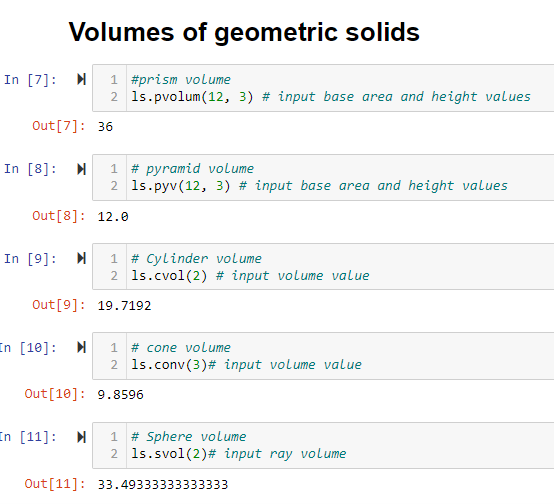top of page
Search

# How to create a PYTHON Library

Updated: Nov 22Author: Leonel Silima

Connect with Leonel

Date of Publication: 30/03/2023

Welcome to another practical article! Well, in this session we will develop a python library capable of the following:

• Perform volume calculations of geometric solids.

• And perform mathematical calculations such as square root, logarithms, potency, and other basic operations such as addition, subtraction, divide, and multiplication.

Without further ado let's look at the steps to follow

## First step: Creating the python library

First, we need to install the python programming language and a text editor. Find in this article the instructions for installing these tools.

## Second step: Project creation

At this point we are going to create a folder where we will store our project and open it with a text editor. In this example I used Vscode but you can use any other editor of your choice. So, inside our text editor we create a file with a py extension where we are going to write our code. In my case the file name is los.py. Below we can see the figure illustrating this point.## Third step: coding

With the project created we can start with the hand in the work. Remember that we only use other libraries when necessary. So, in this project I used math to help me with some mathematical operations and finance to download financial data! Therefore, the following is the coding of this library.## Fourth step: Testing our Library

At this point we test our library. Note that the test file and the library are in the same directory and this is exactly what you should do. In this project the test was carried out in jupyter. However, if you want to use colab for testing, you will need to upload the file to your colab environment.

Though our first step is to import our library into the environment where we want to test it, the procedure is the same as for any python library. First we call the name of the library and then we assign an abbreviation that will be used during its execution. You can see the example below:### Executing the functions

In this first function we want to download data from yfinance as defined in our library. However, we create an object that receives the function to download the data. This happens according to the range and the symbol introduced by the user as seen in the figure below.Now we can also observe the characteristics of the data that we download with the python functions.

• When the market is open• When market is closed• When the price trend is down• When the price trend is high### VOLUME CALCULATIONS OF GEOMETRIC SOLIDS

Another operation included in this library is the calculation of volumes of geometric solids. For this reason, we present the figure of the results obtained for each geometric solid.## Final considerations

We finally realized how amazing the python language is for creating fantastic solutions. Actually, this project was tested on the local machine as it is an educational one. So, in the next session we will learn how to upload it to the pypi platform for online access. Stay tuned for the next article!

Project Finance

Reference C Math